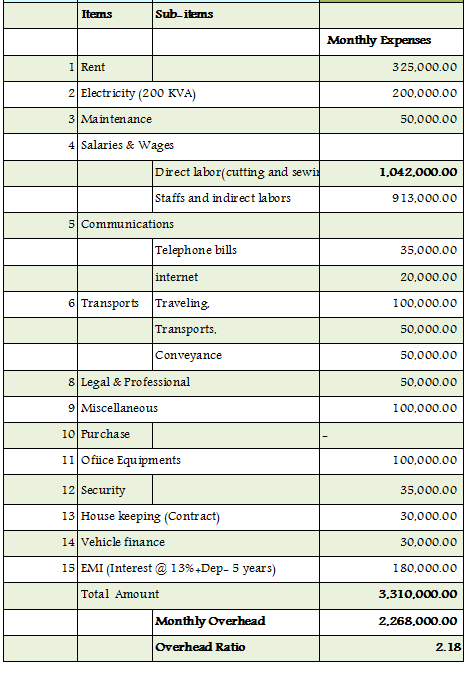Question: I would like to know, how to calculate overheads? Because so many times I have seen that merchants are using a fixed number as overheads. When I ask them how they got this number, they failed to give a satisfactory answer. Please give a brief description. It will be helpful for not only me but also so many new merchants. ... asked by Alok De

When you are making a garment for export orders you should know correct method of costing your product.

Secondly, what are the cost factors to be included to have complete cost?

At the time product cost estimation actual figures are considered for direct material cost and actual direct labor cost. But for the overhead cost, a percentage of direct labor cost (overhead ratio) is considered in costing calculation.

Here is the formula,

Garment manufacturing cost = (Direct Labor cost + Direct Material Cost + Factory Overhead)

Where overhead = x % of direct labor cost

Now the question is how do you find the value of x?

In this article, I have tried to figure it out and explained how you can determine factory overhead ratio.

### What is the overhead cost?

Costs those are not directly related to production volume. No matter how many garments factory produces or sales revenue factory generate, fixed cost to be met each month. These are fixed expenses or indirect cost required to operate a business. For example rent of the factory building, administration cost etc. Two types of overheads are there – fixed factory overhead and variable factory overheads.

### What costs are included under overheads?

List of overheads may vary factory to factory based on organization structure. When you calculate overheads for a production factory only then factory has less overhead. On the other hand, if you calculate overhead of a company having marketing, design, sales and warehouse departments then you will have a lot of other overhead parameters.

Common overheads of a garment factory are like,
• Building rent
• Salary of the staffs and associate payroll costs
• Electricity Bills
• Utilities (Phone bill, internet bills)
• Transport expenses
• Expenses on consumables (Diesel, Chemicals for finishing department)
• Administration cost (salary and travel cost for the managers, CEOs and VPs),
• Employee welfare expenses
• Stationary & printing (indirect material cost)
• Professional and Legal expenses
• Pantry expenses
• House keeping
• Overtime expenses etc.
For the actual list of overheads cost parameters of your factory you can contact to your accountant department.

To calculate factory overhead ratio you have to collect following data from accounts departments for last six months. Fixed factory overheads will be same for all six months but for variable factory overheads take average value to calculate factory overhead ratio.

1. Direct labor wages (A)

So factory overhead over direct labor = B / A

For example, suppose monthly direct labor cost and factory overheads of a small factory are INR 1,042,000.00 and INR 2268,000.00 respectively.

Factory overhead ratio = 2,268,000.00 / 1,042,000.00 = 2.18

An example of the overhead factor calculation sheet is shown in the following table. Note that the ratio may vary from factory to factory. The figure shown below is just for an example.Table: Factory Overheads

### Second Method: Calculation of Factory Overhead per piece:

You can also determine factory overhead per piece using monthly production data or machine productivity figures. Calculate average monthly production of your factory. Divide Monthly factory overheads by monthly production quantity to determine per piece factory overheads. OR calculate monthly production using machine productivity figure.
For example, Suppose machine productivity of the factory (having 150 sewing machines) discussed in above example is 10 pieces per day.
Monthly production of the factory = 150 X 10 X 26 (working days) = 39000 pieces
= ( 2,268,000.00 / 39000 ) = INR 58.15

### How to use overhead ratio in garment cost estimation?

First, calculate labor cost of the garment from garment SAM, line efficiency and labor daily wages. Suppose labor cost per garment is INR 30.00

Calculate overhead amount using overhead ratio and add overhead amount to labor cost and direct material cost to estimate garment manufacturing cost. I am using factory overhead ratio from the above table.

So, overhead cost per garment will be 30 * 2.18 = INR 65.40
Direct material cost is 150.00

Total Cost of the garment = INR (30.00 + 150.00 + 65.40 ) = INR 245.40